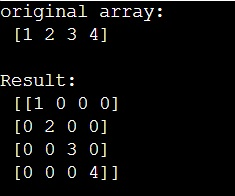# How to convert a column or row matrix to a diagonal matrix?

Learn, how to convert a column or row matrix to a diagonal matrix?
Submitted by Pranit Sharma, on March 13, 2023

## Converting a column/row matrix to a diagonal matrix

Diagonals of an array are defined as a set of those points where the row and the column coordinates are the same.

Suppose that we are given a 1D numpy array that contains a single row. We need to convert this row into a diagonal of a matrix i.e., we want all the elements of this array to be the diagonal of a matrix, and the rest all the elements of the matrix would be zero.

To convert a column or row matrix to a diagonal, we can use the numpy.diag() function. It is used to extract or construct a diagonal array.

Let us understand with the help of an example,

## Python code to convert a column or row matrix to a diagonal matrix

```# Import numpy
import numpy as np

# Creating a numpy array
arr = np.array([1,2,3,4])

# Display original array
print("original array:\n",arr,"\n")

# Creatig a diagonal matrix
res = np.diag(arr)

# Display result
print("Result:\n",res)
```

### OutputLanguages: » C » C++ » C++ STL » Java » Data Structure » C#.Net » Android » Kotlin » SQL
Web Technologies: » PHP » Python » JavaScript » CSS » Ajax » Node.js » Web programming/HTML
Solved programs: » C » C++ » DS » Java » C#
Aptitude que. & ans.: » C » C++ » Java » DBMS
Interview que. & ans.: » C » Embedded C » Java » SEO » HR
CS Subjects: » CS Basics » O.S. » Networks » DBMS » Embedded Systems » Cloud Computing
» Machine learning » CS Organizations » Linux » DOS
More: » Articles » Puzzles » News/Updates